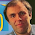## Friday, January 2, 2015

### Triplet Production

In the 4th edition of Intermediate Physics for Medicine and Biology, Russ Hobbie and I describe how x rays interact with tissue by pair production.
A photon with energy above 1.02 MeV can produce a particle–antiparticle pair: a negative electron and a positive electron or positron… Since the rest energy (mec2) of an electron or positron is 0.51 MeV, pair production is energetically impossible for photons below 2mec2 = 1.02 MeV.
One can show, using 0 = pc for the photon, that momentum [p] is not conserved by the positron and electron if [the conservation of energy] is satisfied. However, pair production always takes place in the Coulomb field of another particle (usually a nucleus) that recoils to conserve momentum. The nucleus has a large mass, so its kinetic energy p2/2m is small…
Then we discuss a related process: triplet production.
Pair production with excitation or ionization of the recoil atom can take place at energies that are only slightly higher than the threshold [2mec2]; however, the cross section does not become appreciable until the incident photon energy exceeds 4mec2 = 2.04 MeV, the threshold for pair production in which a free electron (rather than a nucleus) recoils to conserve momentum. Because ionization and free-electron pair production are (γ, eee+) processes, this is usually called triplet production.”Spacetime Physics, by Taylor and Wheeler.
Where does the factor of four in “4mec2” come from? To answer that question, we must know more about special relativity than is presented in IPMB. We know already that the energy of a photon is and the momentum is hν/c, where ν is the frequency, h is Planck’s constant, and c is the speed of light. What we need in addition is that the energy of an electron with rest mass me is γmec2, and its momentum is βγmec, where β is the ratio of the electron’s speed to the speed of light, β = v/c, and γ = 1/sqrt(1−β2). The factors of β and, especially, γ may look odd, but they are common in special relativity. To learn how they arise, read the marvelous book Spacetime Physics by Edwin Taylor and John Archibald Wheeler. Assume a photon with energy interacts with an electron at rest (β = 0, γ = 1). Furthermore (and this is not obvious), assume that after the collision the original electron and the new electron-positron pair all move in the direction of the original photon, and travel at the same speed. The conservation of energy requires
+ mec2 = 3γmec2,

and conservation of momentum implies

hν/c = 3βγmec .

The rest is algebra. Eliminate and you find that 3γβ + 1 = 3γ. Then use γ = 1/sqrt(1−β2) to find that β = 4/5 and γ = 5/3. (I love how the Pythagorean triple 3, 4, 5 arises in triplet production). Then conservation of energy or conservation of momentum implies = 4mec2. Now you know the origin of that mysterious factor of four.

The paper “Pair and Triplet Production Revisited for the Radiologist” by Ralph Raymond (American Journal of Roentgenology, Volume 114, Pages 639–644, 1972) provides additional details. To learn about special relativity, I recommend either Spacetime Physics (their Sec. 8.5 analyzes triplet production) or Space and Time in Special Relativity by one of the best writers of physics, N. David Mermin. I hear Mermin’s recent book It’s About Time is also good, but I haven’t read it yet.

1.Thank you for another great year of Roth's Blog. It's a "must read" for me. Happy 2015!

2.Thanks, but what did you think of the Dec 12 entry?

1.Appreciated the December nerve entries VERY much. Only now putting together comments for your Dec 12, since we've been away. Thank you again.